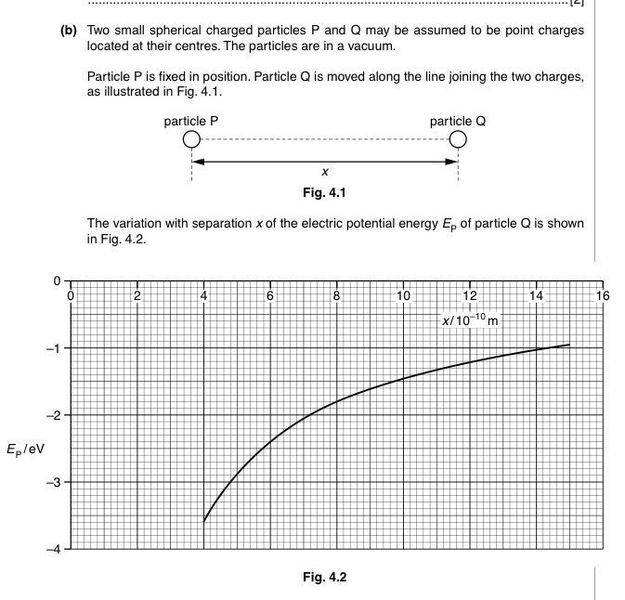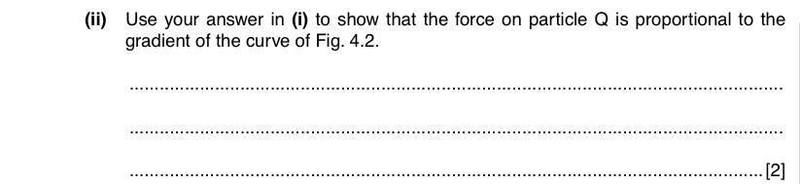# Show that force is proportional to gradient of PE graph

1. Homework StatementOk, so part i) asks us to state how the magnitude of electric field strength is related to potential gradient, and that I answered electric field strength is potential gradient.

## Homework Equations

Electric field strength E=Q/(4πεr^2)
F=Qq/(4πεr^2)
Electric potential energy = Qq/(4πεr)

## The Attempt at a Solution

I know that the electric force is proportional to EPE, but I can't seem to relate to part i)

Electric field strength E=Q/(4πεr^2)
F=Qq/(4πεr^2)
Electric potential energy = Qq/(4πεr)

## The Attempt at a Solution

I know that the electric force is proportional to EPE, but I can't seem to relate to part i)

as your given with a curve giving EPE as varying with r ;you can use the curve to find graphically the electric field/force;
i think you know how to find slope of a curve
- using a tangent drawn at the point chosen by you and the slope of the tangent will give you the rate of change of potential with r.
so you can draw a tangent and check whether you get the same value.
or consult a coordinate geometry book -finding slope of a curve - i think its related to Tan of the angle subtended by the tangent.

haruspex
Homework Helper
Gold Member
1. Homework Statement

View attachment 100658

View attachment 100659
Ok, so part i) asks us to state how the magnitude of electric field strength is related to potential gradient, and that I answered electric field strength is potential gradient.

## Homework Equations

Electric field strength E=Q/(4πεr^2)
F=Qq/(4πεr^2)
Electric potential energy = Qq/(4πεr)

## The Attempt at a Solution

I know that the electric force is proportional to EPE, but I can't seem to relate to part i)
It seems so simple that I'm not sure where your difficulty lies.
You understand how field strength relates to potential gradient; how potential relates to energy?; how potential gradient relates to gradient in a graph of potential?; how field strength relates to force? Which step are you missing?

It seems so simple that I'm not sure where your difficulty lies.
You understand how field strength relates to potential gradient; how potential relates to energy?; how potential gradient relates to gradient in a graph of potential?; how field strength relates to force? Which step are you missing?
I thought that force is EPE gradient. Why do we need to relate to electric field strength and potential gradient to show that force is proportional to the gradient of EPE graph ?

haruspex
Homework Helper
Gold Member
I thought that force is EPE gradient
No. The force depends on the test charge placed in the field. The potential gradient is independent of any test charge.

No. The force depends on the test charge placed in the field. The potential gradient is independent of any test charge.
Ahh I think I get it now. But EPE is dependent on the test charge, am I right?
So, force on Q is proportional to the electric field strength of P, which is the potential gradient. And potential gradient is proportional to the EPE gradient, hence, force on Q is proportional to EPE gradient,right?

haruspex
Homework Helper
Gold Member
Ahh I think I get it now. But EPE is dependent on the test charge, am I right?
So, force on Q is proportional to the electric field strength of P, which is the potential gradient. And potential gradient is proportional to the EPE gradient, hence, force on Q is proportional to EPE gradient,right?
Yes, sorry, I read EPE as electric potential - missed the final E for energy (not anabbreviation I'm familiar with). So you were right, the force is the gradient of the EPE, while the field is the gradient of the EP. And the first pair relates to the second pair by ...

Yes, sorry, I read EPE as electric potential - missed the final E for energy (not anabbreviation I'm familiar with). So you were right, the force is the gradient of the EPE, while the field is the gradient of the EP. And the first pair relates to the second pair by ...
Oh, that's ok. First pair = Second pair x test charge

haruspex
Homework Helper
Gold Member
Oh, that's ok. First pair = Second pair x test charge

Erm, not really..
1) Froce on Q is proportional to field strength of P
3) so force is proportional to the gradient of EPE

why can't I just straight away write :
1+3) Froce on Q is the gradientof EPE

haruspex
Homework Helper
Gold Member
Erm, not really..
1) Froce on Q is proportional to field strength of P
3) so force is proportional to the gradient of EPE

why can't I just straight away write :
1+3) Froce on Q is the gradientof EPE
Because it asked you to derive it from "1. field is gradient of potential":
2. Force = field x charge
3. EPE= potential x charge
So ...

Because it asked you to derive it from "1. field is gradient of potential":
2. Force = field x charge
3. EPE= potential x charge
So ...
(Field x charge)= (potential x charge) / distance

We can't straght away say that force is gradient of EPE, because that isn't by definition, is it?

haruspex
Homework Helper
Gold Member
(Field x charge)= (potential x charge) / distance
No, /distance is not the same as gradient. Gradient is the limit of change/distance as the distance tends to zero.
Using the 1, 2 and 3 that I posted, force = charge x field = charge x gradient of potential = gradient of (potential x charge) = gradient of EPE.
The third step is valid because charge is a constant.
We can't straight away say that force is gradient of EPE, because that isn't by definition, is it?
It's not by definition, but it does follow immediately from ##work=\int \vec F .\vec {ds}##.

No, /distance is not the same as gradient. Gradient is the limit of change/distance as the distance tends to zero.
Using the 1, 2 and 3 that I posted, force = charge x field = charge x gradient of potential = gradient of (potential x charge) = gradient of EPE.
The third step is valid because charge is a constant.

It's not by definition, but it does follow immediately from ##work=\int \vec F .\vec {ds}##.
So basically force is the gradient of EPE graph, right?
Thanks, I think I've got it